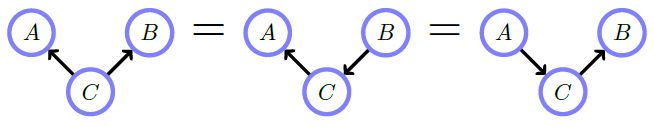Show Question
Math and science::INF ML AI

# Belief networks: independence examples1 a) Marginalizing over $$C$$  makes $$A$$ and $$B$$ independent. In other words, $$A$$ and $$B$$ are (unconditionally) independent $$p(A,B) = p(A)p(B)$$. In the absence of any information about the effect of $$C$$ we retain this belief.1 b) Conditioning on $$C$$ makes A and B (graphically) dependent $$p(A, B \vert C) \ne p(A \vert C)p(B \vert C)$$. Although the causes are a priori independent, knowing the effect $$C$$ can tell us something about how the causes colluded to bring about the effect observed.2. Conditioning on $$D$$, a descendent of a collider $$C$$ makes $$A$$ and $$B$$  (graphically) dependent. $$p(A, B \vert D) \ne p(A \vert D)p(B \vert D)$$3 a) $$p(A, B, C) =$$ $$p(A \vert C)p(B \vert C)p(C)$$. Here there is a 'cause' $$C$$ and independent 'effects' $$A$$ and $$B$$.

3 b) Marginalizing over $$C$$ makes $$A$$ and $$B$$ (graphically) dependent. $$p(A, B) \ne p(A)p(B)$$. Although we don't know the 'cause', the 'effects' will nevertheless be dependent.3 c) Conditioning on $$C$$ makes $$A$$ and $$B$$  independent. $$p(A, B \vert C) = p(A \vert C)p(B \vert C)$$. If you know the 'cause' $$C$$, you know everything about how each effect occurs, independent of the other effect. This is also true for reversing the arrow from $$A$$ to $$C$$—in this case, $$A$$ would 'cause' $$C$$ and then $$C$$ would 'cause' $$B$$. Conditioning on $$C$$ blocks the ability of $$A$$ to influence $$B$$.Finally, these following graphs all express the same conditional independence assumptions.#### Source

Bayesian Reasoning and Machine Learning
David Barber
p42-43